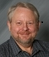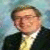## mixture of characters, numerics and special charactersTags:
AS/400
AS/400 Query
i have a string which is a combination of characters,numerics and special characters. how to calculate number of characters,numerics and special characters present in it?

Software/Hardware used:
as400

Thanks. We'll let you know when a new response is added.

Every key (number, special character, or letter) is represented by 8 binary(1s and 0s) digits which the computer use as a lunguage to understand what it is that you want it to do. To count tham use computer firmware will only identify which one is a number and which one is charecter.

## Discuss This Question: 5 Replies

Thanks. We'll let you know when a new response is added.
•Can you give us the business application for this. We may be better able to assist you
report
•You could interpret the string as an array, sort the array, loop through it counting them with a break whenever the character changed. At each break, store the break-character and the count in a second array. Tom
report
•Come to think of it, once the array is sorted, you could use %check() to skip through the resulting string. It could give you unique characters and counts for each one. Tom
report
•Does "characters" include characters from a double byte character set (DBCS)? If so, additional information is needed -- such as datatype (mixed vs graphic).
report
• I am italian, i wrote this one for me, you must yout language character H DECEDIT('0,') DATEDIT(*YMD/) ********************************************************************** * Count Space,Num,AlfaUp,ALfaLo,AlfaSpec characters in a string * ********************************************************************** * * field definition D CountSpac S 6S 0 INZ D CountNum S 6S 0 INZ D CountAlfUp S 6S 0 INZ D CountAlfLo S 6S 0 INZ D CountSpec S 6S 0 INZ D CountTot S 6S 0 INZ d \$msg s 51a d \$rtrn s 1a Inz d \$string_data s 20a d \$scan_data s 1a d \$replace_data s 1a d \$pos s 10i 0 * C *Entry Plist C Parm \$string_data C Clear \$msg C Eval \$msg='Stringa:'+\$string_data C \$msg DSPLY \$rtrn * C Eval CountTot =%Len(%trimr(\$String_data)) * Count Space Character /Free DoW %Scan(' ': %TRIMR(\$string_data)) > *Zeros; \$string_data= %Replace('' : \$string_data: %Scan(' ': %TRIMR(\$string_data)): 1); EndDo; /end-free C Eval CountSpac=CountTot-%Len(%trimr(\$String_data)) C Clear \$msg C Eval \$msg='Stringa:'+\$string_data C \$msg DSPLY \$rtrn C Clear \$msg C Eval \$msg='Space:'+(%EDITC(%Int(CountSpac):'K')) C \$msg DSPLY \$Rtrn * Count Space Character C MOVE '0' \$SCAN_DATA C EXSR RPLALL C MOVE '1' \$SCAN_DATA C EXSR RPLALL C MOVE '2' \$SCAN_DATA C EXSR RPLALL C MOVE '3' \$SCAN_DATA C EXSR RPLALL C MOVE '4' \$SCAN_DATA C EXSR RPLALL C MOVE '5' \$SCAN_DATA C EXSR RPLALL C MOVE '6' \$SCAN_DATA C EXSR RPLALL C MOVE '7' \$SCAN_DATA C EXSR RPLALL C MOVE '8' \$SCAN_DATA C EXSR RPLALL C MOVE '9' \$SCAN_DATA C EXSR RPLALL C Eval CountNum = C CountTot-CountSpac-%Len(%trimr(\$String_data)) C Clear \$msg C Eval \$msg='Stringa:'+\$string_data C \$msg DSPLY \$rtrn C Clear \$msg C Eval \$msg='Numb:'+(%EDITC(%Int(CountNum):'K')) C \$msg DSPLY \$Rtrn * Count Upper Normal Character C MOVE 'A' \$SCAN_DATA C EXSR RPLALL C MOVE 'B' \$SCAN_DATA C EXSR RPLALL C MOVE 'C' \$SCAN_DATA C EXSR RPLALL C MOVE 'D' \$SCAN_DATA C EXSR RPLALL C MOVE 'E' \$SCAN_DATA C EXSR RPLALL C MOVE 'F' \$SCAN_DATA C EXSR RPLALL C MOVE 'G' \$SCAN_DATA C EXSR RPLALL C MOVE 'H' \$SCAN_DATA C EXSR RPLALL C MOVE 'I' \$SCAN_DATA C EXSR RPLALL C MOVE 'L' \$SCAN_DATA C EXSR RPLALL C MOVE 'M' \$SCAN_DATA C EXSR RPLALL C MOVE 'N' \$SCAN_DATA C EXSR RPLALL C MOVE 'O' \$SCAN_DATA C EXSR RPLALL C MOVE 'P' \$SCAN_DATA C EXSR RPLALL C MOVE 'Q' \$SCAN_DATA C EXSR RPLALL C MOVE 'R' \$SCAN_DATA C EXSR RPLALL C MOVE 'S' \$SCAN_DATA C EXSR RPLALL C MOVE 'T' \$SCAN_DATA C EXSR RPLALL C MOVE 'U' \$SCAN_DATA C EXSR RPLALL C MOVE 'V' \$SCAN_DATA C EXSR RPLALL C MOVE 'Z' \$SCAN_DATA C EXSR RPLALL C MOVE 'X' \$SCAN_DATA C EXSR RPLALL C MOVE 'Y' \$SCAN_DATA C EXSR RPLALL C MOVE 'K' \$SCAN_DATA C EXSR RPLALL C MOVE 'J' \$SCAN_DATA C EXSR RPLALL C MOVE 'Z' \$SCAN_DATA C EXSR RPLALL C MOVE 'W' \$SCAN_DATA C EXSR RPLALL C Eval CountAlfUp=CountTot-CountNum-CountSpac C -%Len(%trimr(\$string_data)) C Clear \$msg C Eval \$msg='Stringa:'+\$string_data C \$msg DSPLY \$rtrn C Clear \$msg C Eval \$msg='Upper:'+(%EDITC(%Int(CountAlfUp):'K')) C \$msg DSPLY \$Rtrn * Count Lower Normal Character C MOVE 'a' \$SCAN_DATA C EXSR RPLALL C MOVE 'b' \$SCAN_DATA C EXSR RPLALL C MOVE 'c' \$SCAN_DATA C EXSR RPLALL C MOVE 'd' \$SCAN_DATA C EXSR RPLALL C MOVE 'e' \$SCAN_DATA C EXSR RPLALL C MOVE 'f' \$SCAN_DATA C EXSR RPLALL C MOVE 'g' \$SCAN_DATA C EXSR RPLALL C MOVE 'h' \$SCAN_DATA C EXSR RPLALL C MOVE 'i' \$SCAN_DATA C EXSR RPLALL C MOVE 'l' \$SCAN_DATA C EXSR RPLALL C MOVE 'm' \$SCAN_DATA C EXSR RPLALL C MOVE 'n' \$SCAN_DATA C EXSR RPLALL C MOVE 'o' \$SCAN_DATA C EXSR RPLALL C MOVE 'p' \$SCAN_DATA C EXSR RPLALL C MOVE 'q' \$SCAN_DATA C EXSR RPLALL C MOVE 'r' \$SCAN_DATA C EXSR RPLALL C MOVE 's' \$SCAN_DATA C EXSR RPLALL C MOVE 't' \$SCAN_DATA C EXSR RPLALL C MOVE 'u' \$SCAN_DATA C EXSR RPLALL C MOVE 'v' \$SCAN_DATA C EXSR RPLALL C MOVE 'z' \$SCAN_DATA C EXSR RPLALL C MOVE 'x' \$SCAN_DATA C EXSR RPLALL C MOVE 'y' \$SCAN_DATA C EXSR RPLALL C MOVE 'K' \$SCAN_DATA C EXSR RPLALL C MOVE 'j' \$SCAN_DATA C EXSR RPLALL C MOVE 'z' \$SCAN_DATA C EXSR RPLALL C MOVE 'w' \$SCAN_DATA C EXSR RPLALL C Eval CountAlfLo=CountTot-CountNum-CountAlfUp C -CountSpac-%Len(%trimr(\$String_data)) C Clear \$msg C Eval \$msg='Stringa:'+\$string_data C \$msg DSPLY \$rtrn C Clear \$msg C Eval \$msg='Lower:'+(%EDITC(%Int(CountAlfLo):'K')) C \$msg DSPLY \$Rtrn * Count Special Character C Eval CountSpec =%Len(%trimr(\$String_data)) C Clear \$msg C Eval \$msg='Stringa:'+\$string_data C \$msg DSPLY \$rtrn C Clear \$msg C Eval \$msg='Special:'+(%EDITC(%Int(CountSpec):'K')) C \$msg DSPLY \$Rtrn C SETON LR * C RPLALL BEGSR /Free DoW %Scan(\$scan_data: %TRIMR(\$string_data)) > *Zeros; \$string_data= %Replace('':\$string_data: %Scan(\$scan_data : %TRIMR(\$string_data)): 1); EndDo; /end-free C ENDSR Bye
report